# How to Size a Moisture Separator

## Charts, formulas and web-based calculators for separator sizing

Our article “Understanding Sizing Factors for Centrifugal Separators” described what a centrifugal separator is, the key design criteria required for proper sizing and how to use our web-based sizing tools.  In this article we explain the sizing charts and formulas of which the web-based calculators are based upon.  The two articles combined enable you to both properly size a centrifugal vortex separator and compare performance criteria of liquid removal and pressure drop amongst several separator designs.

We use two charts for sizing separators, one for steam and another for all other gases.  We will review sizing for steam applications first because it is more simplistic.

Over 50 years ago physical testing was performed in a laboratory using steam and compressed air to optimize the efficiency of centrifugal separators.  The test results were plotted on graphs to be used for sizing separators and we have since derived formulas from those performance curves to enable creation of our web-based sizing tools.  These charts and calculators indicate the maximum capacity for removal of droplets and particles larger than 10 microns with 99% efficiency.

The sizing charts have a X, Y and secondary Y axis.  The X axis lists the mass flow rate of steam or the volumetric flow rate for air depending upon the chart.  The Y axis lists absolute pressure (PSIa) and the secondary Y axis the corresponding differential pressure (∆P).  The diagonal lines across the chart are the performance curves for standard separator sizes.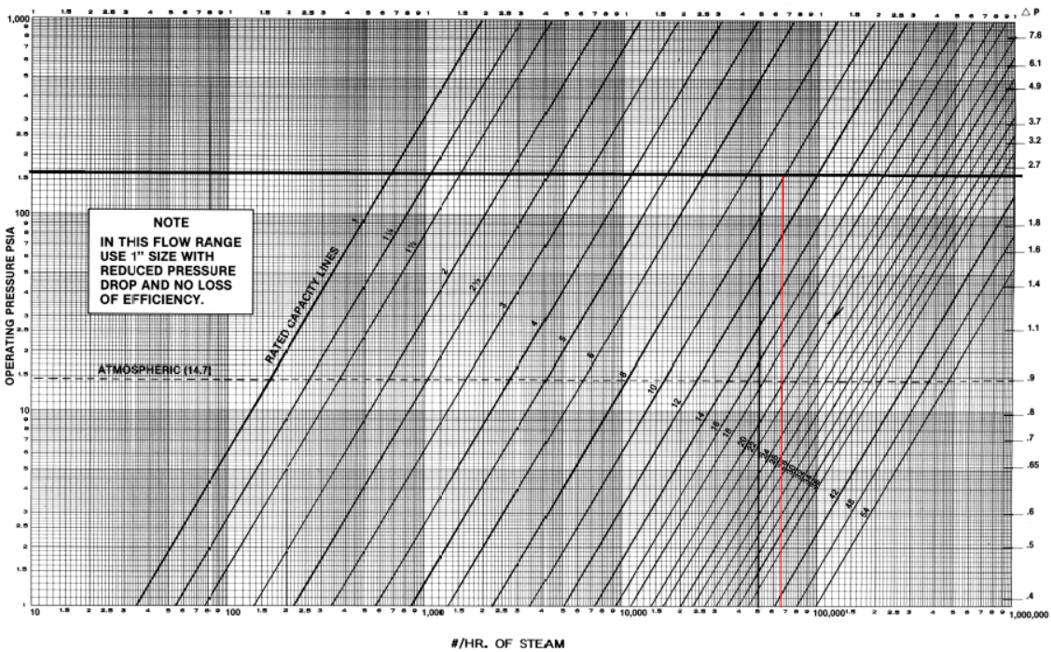Moisture Separator Steam Sizing Chart
The first step is to draw a horizontal line from the design (maximum) PSIa across to the differential pressure (secondary Y axis).

Next draw a vertical line from your design (maximum) flow rate of steam in lbs/hr units.  The separator size required is indicated by the line to the right of where your two lines intersect.

Now draw a vertical line from the intersection of horizontal line and the required separator size line; where it intersects the X axis is the maximum capacity in lbs/hr for that size separator at that specific operating pressure.

To calculate the corresponding differential pressure for your application, use the following formula: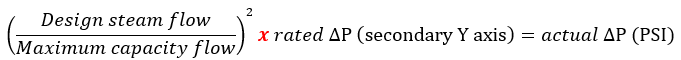Since centrifugal vortex separators have an infinite turndown ratio they maintain their separation efficiency at lower pressures associated with start-up and shut-down.

If you require a lower differential pressure, rewrite the equation above to solve for maximum capacity flow, find that value on the X axis and draw a vertical line upwards to intersect with the PSIa line; the required separator size to satisfy your specific ∆P requirements is the separator size to the right of that intersection.

The full-size steam sizing chart is hyperlinked to the image on this page and it has a sizing example written on it already.

Moisture Separator Sizing for Air and Other Gasses

The procedure for sizing air and other gas applications is the same with respect to drawing horizontal and vertical lines, the difference is that the volumetric air flow needed to use the chart is the “Equivalent Air Flow in SCFM (Qc)”.

Earlier I mentioned testing was performed many years ago using air and the corresponding datapoints formed the basis of separator sizing.  The temperature of the air used for testing was 60°F.  Therefore, if your application happens to be for air at 60°F and you have the maximum flow rate in SCFM, you can go right to the chart.  If the air is at another temperature, you have the mass flow or if it is a gas having a molecular weight different than air (29 MW) then some additional calculations are required before you can use the chart.  These calculations result in Qc “equivalent air flow SCFM”.

The formula for Qc is:  Qc = Qsg x Fg x Ft
Qsg is the rate of flow in CFM
Fg is a correction factor for specific gravity (sg)
Ft is a correction factor for temperature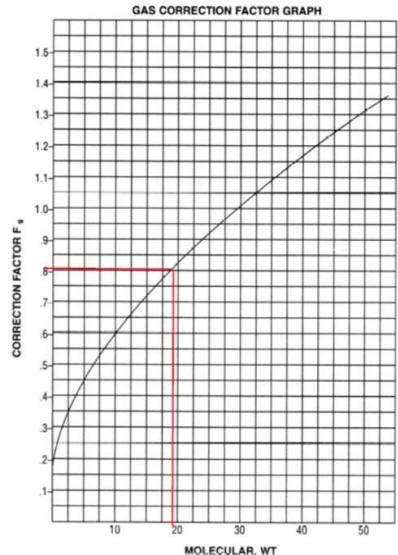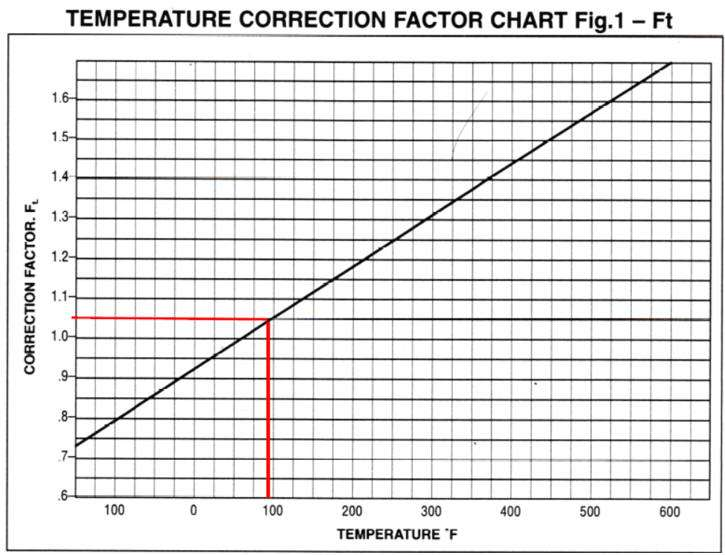We have tables and graphs for Fg and Ft and from those we have derived formulas. There are several ways to calculate Qsg, the easiest is probably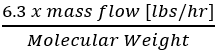.  If your gas is a composition of several different gasses you will need to calculate the equivalent MW using the percentage of each gas in your composition.

Let’s walk through an example for natural gas consisting of 75% Methane (CH4: 16 MW) and 25% Nitrogen (N2: 28 MW).  The design (maximum) pressure is 120 PSIa and the temperature is 90°F.  Our design (maximum) flow rate is 1,000 lbs/hr.

The MW for our calculations is 19 (75% of 16 + 25% of 28). And thus Qsq=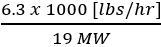= 331.58 CFM.

We have a table specifying Fg for Natural Gas is 0.788 and if you use the graph it would seem to be 0.8; when we have a value in table format we will use that instead of interpolating from a graph; our calculators use a formula.

Next is Ft, and we again have both a table and graph to consult; the table specifies Ft = 1.034 and the graph Ft = 1.05, so we’ll go with the table. Now we can calculate Qc = 331.58 x 0.788 x 1.034 = 270.16 SCFM.

The intersecting lines indicate a 1½” separator is required and its maximum flow rate is 350 SCFM with a rated ∆P of 1.2 PSI.  Thus the actual differential pressure is calculated as 0.715 ∆P.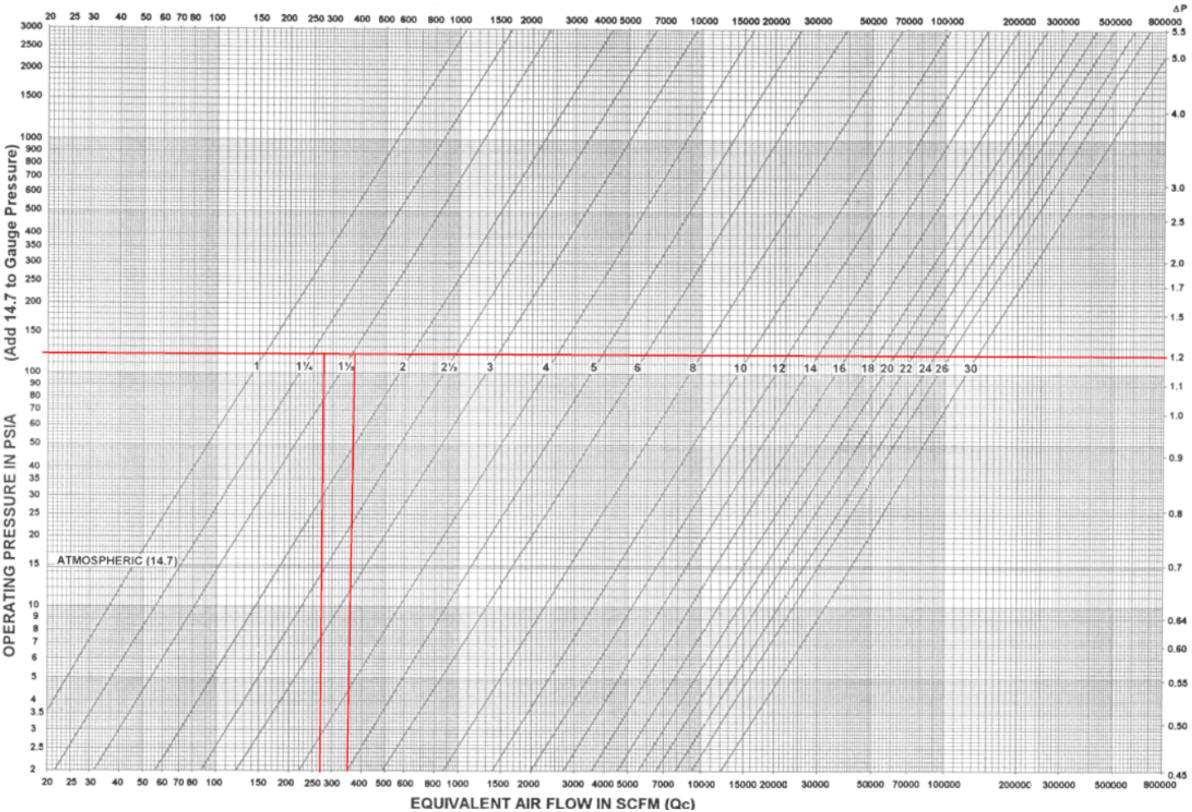Selecting the Best Separator Design
Now that you know the size of the separator you will need to determine which model suits your application the best.

Additional criteria to consider include the volume of condensate to remove, your piping size and orientation, droplet size to remove and physical space available to install the separator.  This aspect of separator selection is described in our article “Efficiency of Centrifugal Vortex Separators”.

Our web-based sizing tool automatically calculates Qc and both rated and actual ∆P.  In addition, it provides the liquid removal rate for all size separator designs.  You can also toggle the size to adjust the liquid removal rate and actual ∆P to quickly determine the separator size and model for your application.  If you have several applications or want to quickly see the effect of changing a variable such as pressure, the online sizing tool is invaluable.

Although we have provided you with the fundamental information and tools to properly size a centrifugal separator for your application, we are here to assist you!  Please contact us by phone, email or web-based inquiry form so we can put our experience to work for you!